Add & Subtract Within 100 Math Video for Kids | K, 1st & 2nd Grade
1%
It was processed successfully!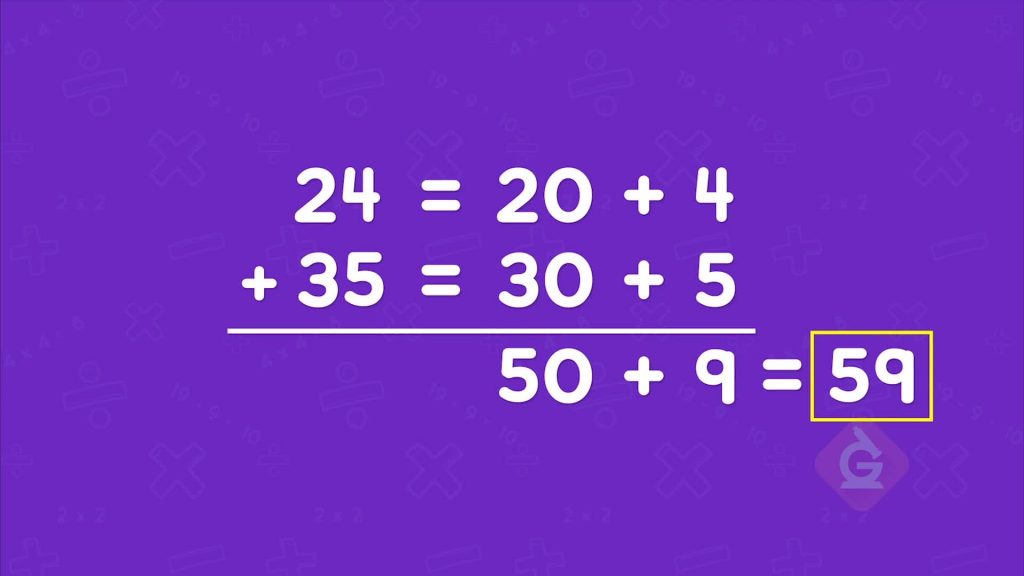WHAT IS ADDING & SUBTRACTING WITHIN 100?

You can break apart a number using place value to help you add. 24 is 2 tens and 4 ones. 24 = 20 + 4. When you break apart two numbers, you can add the tens then add the ones.

To better understand Adding & Subtracting within 100…

WHAT IS ADDING & SUBTRACTING WITHIN 100?. You can break apart a number using place value to help you add. 24 is 2 tens and 4 ones. 24 = 20 + 4. When you break apart two numbers, you can add the tens then add the ones. To better understand Adding & Subtracting within 100…

## LET’S BREAK IT DOWN!

### Place-Value Blocks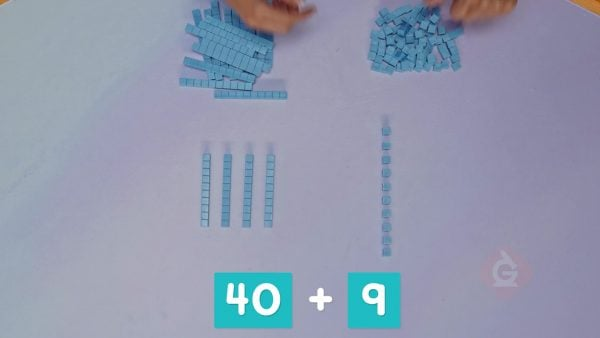Place value is the value of a digit based on its position in a number. The 4 in 49 represents 4 tens. The 9 represents 9 ones. 49 = 40 + 9. You can use place-value blocks to show 4 tens and 9 ones. Erika adds 1 more ones block to the pile. Now there are 10 ones. 10 ones is the same as 1 ten. Now there are 5 tens. 5 tens = 50. Try this one yourself: How can you represent 68 with place-value blocks?

Place-Value Blocks Place value is the value of a digit based on its position in a number. The 4 in 49 represents 4 tens. The 9 represents 9 ones. 49 = 40 + 9. You can use place-value blocks to show 4 tens and 9 ones. Erika adds 1 more ones block to the pile. Now there are 10 ones. 10 ones is the same as 1 ten. Now there are 5 tens. 5 tens = 50. Try this one yourself: How can you represent 68 with place-value blocks?

### Candy Apples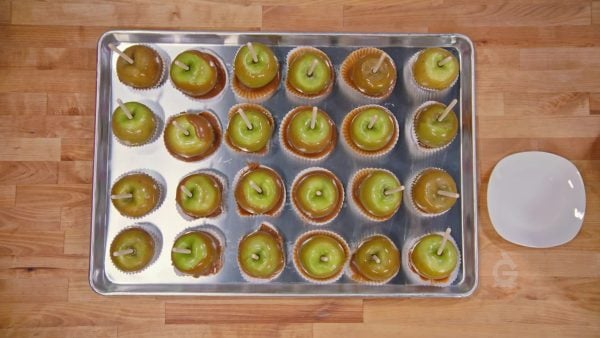Erika has dipped 24 candy apples. Dane has dipped 35. How many candy apples have they dipped in all? Find 24 + 35. 24 is 2 tens and 4 ones. 35 is 3 tens and 5 ones. Add the ones and add the tens. 20 + 30 = 50. 4 + 5 = 9. The total is 50 + 9 = 59. Try this one yourself: Erika has dipped 33 candy apples. Dane has dipped 41 candy apples. How many have they dipped in all?

Candy Apples Erika has dipped 24 candy apples. Dane has dipped 35. How many candy apples have they dipped in all? Find 24 + 35. 24 is 2 tens and 4 ones. 35 is 3 tens and 5 ones. Add the ones and add the tens. 20 + 30 = 50. 4 + 5 = 9. The total is 50 + 9 = 59. Try this one yourself: Erika has dipped 33 candy apples. Dane has dipped 41 candy apples. How many have they dipped in all?

### FlowersThere are 58 blue flowers and 37 red flowers. How many flowers are there in all? Find 58 + 37. 58 is 5 tens and 8 ones. 37 is 3 tens and 7 ones. 50 + 30 = 80. 8 + 7 = 15. Altogether there are 8 tens and 15 ones. Regroup 10 ones as 1 ten. That makes 9 tens and 5 ones, or 95 in all. Try this one yourself: There are 48 yellow flowers and 36 purple flowers. How many flowers are there in all?

Flowers There are 58 blue flowers and 37 red flowers. How many flowers are there in all? Find 58 + 37. 58 is 5 tens and 8 ones. 37 is 3 tens and 7 ones. 50 + 30 = 80. 8 + 7 = 15. Altogether there are 8 tens and 15 ones. Regroup 10 ones as 1 ten. That makes 9 tens and 5 ones, or 95 in all. Try this one yourself: There are 48 yellow flowers and 36 purple flowers. How many flowers are there in all?

### Plastic Bricks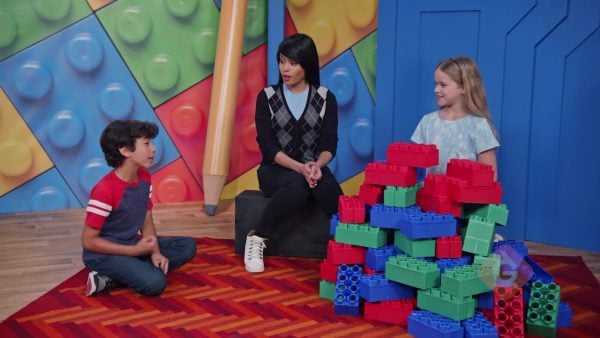Erika has 65 blocks. She gives Dane 41 blocks. How many blocks does Erika have left? Find 65 – 41. 65 is 6 tens and 5 ones. 41 is 4 tens and 1 one. Subtract by place value. 60 – 40 = 20. 5 – 1 = 4. The answer is 2 tens and 4 ones, or 24. Erika has 24 bricks left. Try this one yourself: Erika has 87 blocks. She gives Dane 35 blocks. How many blocks does Erika have left?

Plastic Bricks Erika has 65 blocks. She gives Dane 41 blocks. How many blocks does Erika have left? Find 65 – 41. 65 is 6 tens and 5 ones. 41 is 4 tens and 1 one. Subtract by place value. 60 – 40 = 20. 5 – 1 = 4. The answer is 2 tens and 4 ones, or 24. Erika has 24 bricks left. Try this one yourself: Erika has 87 blocks. She gives Dane 35 blocks. How many blocks does Erika have left?

### Ant Farm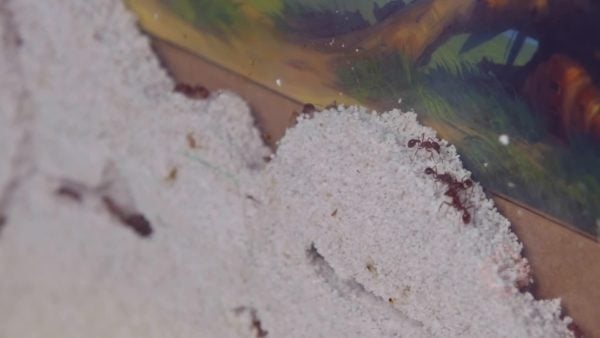Dane has 71 ants in his ant farm. He gives Erika 38 ants. How many ants does Dane have left? Find 71 – 38. 71 is the same as 70 + 1. 38 is the same as 30 + 8. Try to subtract the ones. You can't take away 8 ones from 1 one. Regroup 1 ten in 71 as 10 ones. So 70 = 60 + 11. Subtract the ones. 11 – 8 = 3. Subtract the tens. 60 – 30 = 30. The difference is 30 + 3 = 33. Dane has 33 ants left. Try this one yourself: Dane has 82 worms. He gives Erika 49 worms. How many worms does Dane have left?

Ant Farm Dane has 71 ants in his ant farm. He gives Erika 38 ants. How many ants does Dane have left? Find 71 – 38. 71 is the same as 70 + 1. 38 is the same as 30 + 8. Try to subtract the ones. You can't take away 8 ones from 1 one. Regroup 1 ten in 71 as 10 ones. So 70 = 60 + 11. Subtract the ones. 11 – 8 = 3. Subtract the tens. 60 – 30 = 30. The difference is 30 + 3 = 33. Dane has 33 ants left. Try this one yourself: Dane has 82 worms. He gives Erika 49 worms. How many worms does Dane have left?

## ADD & SUBTRACT WITHIN 100 VOCABULARY

Place value
The value of a digit based on its location in a number.
To bring two or more numbers (or things) together to make a new total.
Subtract
To take one number away from another.
Sum
Difference
The result of subtracting.
Expanded Form
A form of a number that shows a number as the sum of the value of its digits. For example, 234 = 200 + 30 + 4.
Exchanging 10 ones for 1 ten, or 1 ten for 10 ones.

## ADD & SUBTRACT WITHIN 100 DISCUSSION QUESTIONS

I can break apart numbers by their place values, and then add the separate place values together. For example, 82 = 80 + 2. 17 = 10 + 7. 80 + 10 = 90. 2 + 7 = 9. The final sum is 90 + 9 = 99.

### When do you need to regroup when adding 2-digit numbers? How do you regroup?

I need to regroup if I add the ones and the number is 10 or greater. I regroup 10 ones as 1 ten.

### Find 26 + 12. Do you need to regroup?

26 + 12 = 38. 20 + 10 = 30. 6 + 2 = 8. There are only 8 ones, so I don’t need to regroup.

### When do you need to regroup when subtracting 2-digit numbers? How do you regroup?

I need to regroup if the number of ones I need to subtract is greater than the number of ones I'm subtracting from. I regroup 1 ten from the first number as 10 ones. For example, 34 – 29. I regroup 1 ten from 34 as 10 ones. So 34 = 20 + 14. Then I subtract the ones and the remaining tens.

### Find 34 – 29. Do you need to regroup?

34 is 3 tens and 4 ones. 29 is 2 tens and 9 ones. 9 ones are more than 4 ones, so I need to regroup. 34 = 20 + 14. Subtract the ones. 14 – 9 = 5. Subtract the tens. 20 – 20 = 0. The answer is 5.
X

## Success

We’ve sent you an email with instructions how to reset your password.
Ok